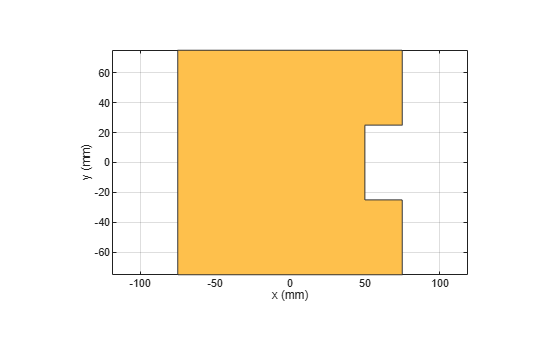# antenna.Rectangle

Create rectangle centered at origin on X-Y plane

## Description

Use the `antenna.Rectangle` object to create a rectangle centered at the origin and on the X-Y plane. You can use `antenna.Polygon` to create single-layer or multi-layered antennas using `pcbStack`.

## Creation

### Syntax

``rect = antenna.Rectangle``
``rect = antenna.Rectangle(Name=Value)``

### Description

example

````rect = antenna.Rectangle` creates a rectangle centered at the origin and on the X-Y plane.```

example

````rect = antenna.Rectangle(Name=Value)` sets properties using one or more name-value pair. `Name` is the property name and `Value` is the corresponding value. You can specify several name-value arguments in any order as `Name1`= `Value1`, `...`, `NameN`=`ValueN`. Properties not specified retain their default values.```

## Properties

expand all

Name of rectangle, specified as a string or a character vector.

Example: `"Rect1"`

Data Types: `char` | `string`

Cartesian coordinates of center of rectangle, specified as a 2-element vector with each element measured in meters.

Example: `[0.006 0.006]`

Data Types: `double`

Rectangle length, specified as a scalar in meters.

Example: `2`

Data Types: `double`

Rectangle width, specified as a scalar in meters.

Example: `4`

Data Types: `double`

Number of discretization points per side, specified as a scalar.

Example: `16`

Data Types: `double`

## Object Functions

 `add` Boolean unite operation on two shapes `subtract` Boolean subtraction operation on two shapes `intersect` Boolean intersection operation on two shapes `plus` Shape1 + Shape2 `minus` Shape1 - Shape2 `and` Shape1 & Shape2 `area` Calculate area of shape in square meters `show` Display antenna, array structures or shapes `plot` Plot boundary of shape `mesh` Mesh properties of metal, dielectric antenna, or array structure `rotate` Rotate shape about axis and angle `rotateX` Rotate shape about x-axis and angle `rotateY` Rotate shape about y-axis and angle `rotateZ` Rotate shape about z-axis and angle `translate` Move shape to new location `scale` Change the size of the shape by a fixed amount `mirrorX` Mirror shape along X-axis `mirrorY` Mirror shape along Y-axis `removeHoles` Remove holes from shape `removeSlivers` Remove sliver outliers from boundary of shape

## Examples

collapse all

Create a rectangle shape using antenna.Rectangle and view it.

`r1 = antenna.Rectangle`
```r1 = Rectangle with properties: Name: 'myrectangle' Center: [0 0] Length: 1 Width: 2 NumPoints: 2 ```
`show(r1)`Create and view a rectangle with a length of 2 m and a width of 4 m.

```r2 = antenna.Rectangle(Length=2,Width=4); show(r2) axis equal```Rotate the rectangle.

`rotateZ(r2,45);``show(r2)`Create a rectangle with a length of 0.15 m, and a width of 0.15 m.

`r = antenna.Rectangle(Length=0.15,Width=0.15);`

Create a second rectangle with a length of 0.05 m, and a width of 0.05 m. Set the center of the second rectangle at half the length of the first rectangle r.

`n = antenna.Rectangle(Center=[0.075,0],Length=0.05,Width=0.05);`

Create and view a notched rectangle by subtracting n from r.

```rn = r-n; show(rn)```Calculate the area of the notched rectangle.

`area(rn)`
```ans = 0.0212 ```

## Version History

Introduced in R2017a